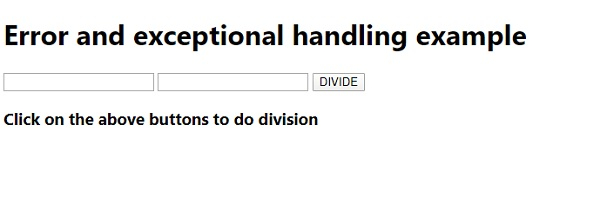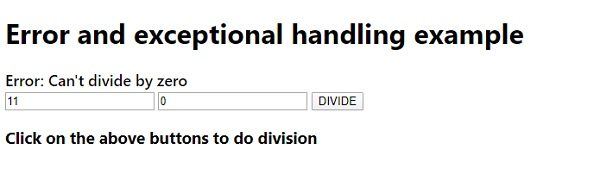# JavaScript Error and Exceptional Handling with Example

Let us see an example to implement error and exception handling concepts with JavaScript −

## Example

Live Demo

<!DOCTYPE html>
<html lang="en">
<meta charset="UTF-8" />
<meta name="viewport" content="width=device-width, initial-scale=1.0" />
<title>Document</title>
<style>
body {
font-family: "Segoe UI", Tahoma, Geneva, Verdana, sans-serif;
}
.sample {
font-size: 18px;
font-weight: 500;
}
</style>
<body>
<h1>Error and exceptional handling example</h1>
<div class="sample"></div>
<input class="inputNum" type="number" />
<input class="inputNum" type="number" />
<button class="Check">DIVIDE</button>
<h3>
Click on the above buttons to do division
</h3>
<script>
let sampleEle = document.querySelector(".sample");
let num, num1;
num = document.querySelectorAll(".inputNum").value;
num1 = document.querySelectorAll(".inputNum").value;
try {
if (num1 === "0") throw "Can't divide by zero";
sampleEle.innerHTML = ${num} divide by${num1} = \${num / num1};
} catch (e) {
sampleEle.innerHTML = "Error: " + e;
}
});
</script>
</body>
</html>

## OutputOn entering the second number as 0 and clicking DIVIDE −